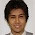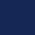## Pages

### Kelly Criterion

Kelly Criterion [Kelly 1956] permits the investor to maximise return on investment, geometrically. The theory was developed by John L Kelly (pictured left) out of the information theory work of Claude Shannon. The Kelly Criterion uses the analogy of a crooked gambler who has control of a private wire (this theory is from the 1950s hence the old terminology) to their local bookmaker.

The wire provides the bookmaker with results. Having control of the wire gives the gambler time to learn the result of a horse race and delay the result getting to the bookmaker long enough to place the winning bet. Obviously, if the wire is perfect you would bet your house on the result but what if the wire had an intermittent fault, how much do you then wager?

The answer is that you bet the size of your edge. If your wire gives you a 100% edge over the bookmaker then you bet 100% of of your bankroll but if you only have a 5% edge then you only bet 5%. No matter what the edge, so long as it is positive, your bankroll will grow geometrically. If your edge is negative then simply lay instead of backing the proposition.

The fraction to bet is given by the equation

where f is the fraction of your bankroll to bet, b is the net money returned on a £1 bet eg. if the odds are 2.1 on Betfair then the return is 1.1, p is the probability of winning and q is the probability of losing (1 - p).

For example, a fair bookie offers 2.0 on either tossing heads or tails. However, the coin is biased to heads with probability 0.6. You must therefore bet ((1 * 0.6) - 0.4) /1 = 0.2 (20% of your bankroll) on heads. Some people prefer to use a fractional Kelly bet as a full Kelly can make your bankroll fluctuate considerably. A Half Kelly, in the previous example, would have you betting 10% rather than 20%.

Model Kelly Criterion in a spreadsheet

You can model geometric growth with Kelly Criterion using a spreadsheet.

Click on the image above to see the detail.

In cell B6 we see the equation for the size of Kelly bet as a fraction of the total bankroll. The figures above are fixed values for predicted probabilities and return. In cells B5 and B7 we see variations of the Kelly Criterion; f/2 is a fractional Kelly bet of one half of the recommended amount. 2f is double the recommended amount.

Betting above the recommended Kelly Criterion will result in ruin. Betting a fractional amount of the Kelly Criterion may be wise if your system is inaccurate or open to fluctuations. A long losing streak will ruin a bankroll, even if using Kelly Criterion, so always consider optimising a fractional Kelly bet.

In the sample run we see a starting bankroll of 100, a wager given B6 multiplied by B10, a probability given by the random function in the range 0 to 1 and remainder being the bankroll updated with the win or the loss. As you can see, the bankroll takes time to ramp up but ramp up it does. Of course, in reality such ramping up will run into market capacity problems where your bet size is too big to be accepted.

Reference

Kelly, J., (1956), "A New Interpretation of the Information Rate", Bell System Technical Journal 1, pp. 917–926.

Try different fractions of Kelly Criterion to see what effect they have on the bankroll.

To read more on the history of Kelly Criterion read Fortune's Formula

1.2.3.Hi James, Im trying to work out if and what fraction of the Kelly Criterion I should use based on the following:

59% SR over a sample size of 91 trades
Average odds of 10.00
Bankroll: £100

Any help appreciated. Thanks Michael

1.Strike Rate is a percentage. To convert a percentage to a probability divide by 100.

The rest is as above. It will be a good exercise for you now and for the future.

This is just A-level standard, which you will need to become a beginner quant-trader.

2.After calculating your fraction tell me why you wouldn't bet that amount and what might be wrong with your model.

4.Because its a relatively small sample size (91 trades) so variance may play its part and wipe my roll out relatively quickly staking 37% of my roll on one trade seems excessive. I check my longest losing run (9 trades) but more often or not its 2-4.

My model is based on my subjective opinion which is a concern. I've tried to systemize my process but due to the nature of horse racing I'm finding it rather difficult to do so.

5.Thank you for your advice James. So level stakes then? I'm returning 68.3pts net profit based on that sample. Square root staking has been recommended to me previously not sure your thoughts on that.

1.You have me at 6s and 7s. I've three jobs on at the same time.

Your Kelly fraction should be 54%

bp-q/b = ((9*.59)-.41)/9

b = odds - 1, p = prob win, q = prob lose

Still a large figure.

A fractional Kelly would make more sense. Divide by 2 or 4 or whatever.

I would say you have some large odds in your sample that are skewing your average odds.

If I were you, forget Kelly, it would give you a heart attack if your bankroll is just £100. Add lots of zeroes before you get involved with large trades.

6.Hi James, thought I would share my little experiment based on a fractional use of Kelly C (20%)

Over my sample size of 91 trades with a starting bankroll on just £40. My worst losing run saw me still in profit to the tune of £8. My roll (in theory) would finish in profit to the tune of £5027.60. Placing individual trades (in theory) with a stake size upto £338.13!

I see why the formula scares the hell out of alot of traders. It requires absolute precision and clarity about your edge. But I think its masterful how it works.

7.An interesting topic. The reason your stakes are so large is you have over a 50% strike rate at odds of 10! You have a 54% edge compared to 1 to 5% usually seen.
The main problem with using Kelly is most people ignore the denominator (divide by average odds) as most explanations are given for low odds examples (as above), around 2 so the denominator is close to 1 and redundant.
The other practical problem with using the formula is that it is based on future edge, which in sports betting can only be guessed at.
The problem with level stakes is not that you may be under staking the geometric optimum growth profile but that you will probably over stake by ignoring the divide by average odds. This is hidden for low odds bettors but is a killer at your odds.
With your £100 bank, assume your edge is far less, say 10% (still exceptional and therefore a risky assumption) and your Kelly stake is £1 (edge divided by odds).
As your database grows you should get more confidence in your likely edge calculation.
I stake Kelly (automatically through the programme I wrote based on James' excellent book "Programming for Betfair") and I found the easiest implementation was to cap Kelly at some maximum. So Kelly at high odds (where you need it) and level at low odds (to avoid the eye-watering variation in stakes that Kelly can produce).
The other point to remember is if you bet a portfolio of systems you can't Kelly stake them all, so a practical cap that reflects the edge of the portfolio as a whole is the way ahead.

Regards